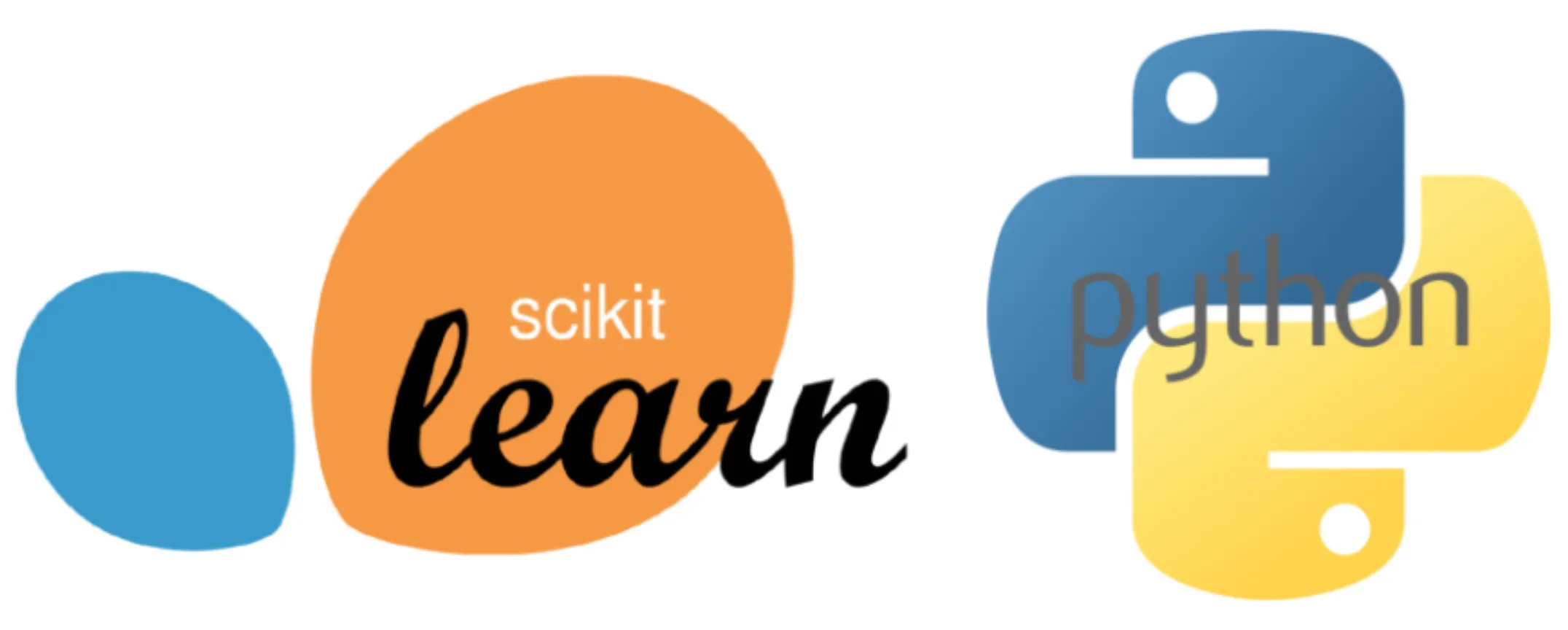# sklearn

## What is Scikit-learn?

Scikit-learn, also known as sklearn, is an open-source, machine learning and data modeling library for Python. It features various classification, regression and clustering algorithms including support vector machines, random forests, gradient boosting, k-means and DBSCAN, and is designed to interoperate with the Python libraries, NumPy and SciPy.

Scikit-learn was first released in 2010, and it has since gained a prominent place in the Python machine learning ecosystem. It implements numerous data modeling and machine learning algorithms, and provides consistent Python APIs. It supports a standardized and concise model interface across models. For example, Scikit-learn makes use of a simple fit/predict workflow model for its classification algorithms.Scikit-learn integrates well with many other Python libraries, such as matplotlib and plotly for plotting, NumPy for array vectorization, Pandas dataframes, SciPy, and many more. You can pass NumPy arrays and Pandas dataframes directly to Scikit-learn’s algorithms.

It provides a comprehensive set of supervised and unsupervised learning algorithms, covering areas such as:

• Classification - Identifying which category an object belongs to.
• Regression - Predicting a continuous-valued attribute associated with an object.
• Clustering - Automatic grouping of similar objects into sets, with models like k-means.
• Dimensionality Reduction - Reducing the number of attributes in data for summarization, visualization and feature selection, with models like Principal Component Analysis (PCA).
• Model Selection - Comparing, validating and choosing parameters and models.
• Pre-processing - Feature extraction and normalization, including defining attributes in image and text data.

Scikit-learn is largely written in Python, and uses NumPy extensively for high-performance linear algebra and array operations. Some core algorithms are written in Cython to improve performance.